## Introduction

With data that have time-related information, time features can be created to to possibly add more information to the models.

Since how to consider time series for machine learning is a broad topic, this article only aims to introduced basic ways to create time features for those models.

### Type of data that is expected for this application

It is expected that transaction data type or any kind that similar to it will be the common one for this application. Other kinds of data that have timestamps information for each data points should also be applicable to some extent of this approach well.

### Considering before attempt: a need ofanalyzing the problem and scope

For data with time element, it can be presented as a time series, which is how a set of data points described an entity are follow ordered indexes of time. One aspect considered for time series is that observations is expected to depend on previous one in time sequence, with the later is correlated to the one before. In those cases, using time series models for forecasting is a straightforward approach to use this data. Another way to approach it is to use feature engineering to transform data to have features that can be used for supervised machine learning model, which is the focus of this article.

Using time series model or adapting machine learning model is depended. In some cases, domain knowledge or business requirement will influence this decision.  It is better to analyze the problem first to see the need of using either one or both type of models.

Regardless of the domain knowledge  or business requirement aspects, the approach decision should have always considering the efficiency the approach will bring for in term of accuracy and computation cost.

## Basic methods

### A first preprocessing step to have a first set of time features: extracting time information from timestamp

The most straightforward thing to do is to extract basic time units, which for instance are hours, date, month, year into separates features. Another kind of information that can also be extracted is the characteristic of the time period, which could be whether the time is at a part of days (morning, afternoon), is weekend or not or is it a holiday, etc.

In some business requirements or domains’ aspects, those initial features at this level is already needed to see if the value of observation is follow those factors or not. For example, the data is the record of timestamps of customer visiting a shop and their purchase. There is a need to know at which hours, date, month… a customer would come and purchase so that follow up actions can be made to increase sales.

### Aggregate techniques

Regarding feature engineering for time data, the well-known technique that commonly used is aggregate features by taking statistics (variance, max, min, etc.) of the set of values grouped by a desired time unit: hours, days, months…

Apart from that, a time window could be defined and compute aggregate by rolling or expanding time window.

• Rolling: have a fixed time window size and to predict a value for the data point at a time, features will be computed from aggregating backward number of time steps according to the time window
• Expanding: from the data point, the window will be the whole record of past time steps.

There are also two aspects of aggregating:

• Aggregating to create new features for the current data points. For the first case, the model is considered to include the time series characteristic, meaning a moment will likely to be related by other moments from the recent past.
• Aggregating to create a new set of data points with corresponding new set of features from the current ones. For the second one, the new number of data points considering for the model is changed and each new data point is the summary of information from a subset of initial data points. As a results, objects for the models may be shifted like being mentioned in considering before part. If the data only about information record of one entity, or in other word only contains one time series of an entity, through this techniques, the new computed data points can be the summary of other features’ value in the chosen time unit. On the other hand, if there are more entities observed in the data set, each new data points is then the summary information of each observed entities.

How to decide on the focus objects for the problem and the approach is situational but for fresh problem and fresh data with no specific requirement or prior domain knowledge, it is better to consider all of them for the model and execute feature selection to see if the created time features is of any value.

## Dealing with hours of a day – Circular data

For some needs, specific time of a day is required to be focus at. A use case of of detecting fraud transactions is a good example for this. To find something like the most frequent time that a kind of behaviour is performed for instance, using arithmetic mean mean may be misleading and is not a good representation. An important point need to be considered is that hours of day is a circular data and it should be represented on a circular axis with its’ value between 0 to 2π. To have a better representation of the mean, using von Mises distribution to have periodic mean is a suitable approach for this situation (Mishtert, 2019).

## Validation for the model

Before the model building, a validation set is needed to be selected from the data first. In the usual cases, to avoid overfitting data will be randomly shuffled and then will be divided into training set and validation set. However, for this kind of situations it shouldn’t be done so to avoid the mistake of having past data in the validation and the future data in the training, in other words using future data to predict the past.

## Definition

In computer science, most of algorithms run to completion: they provide a single answer after performing some fixed amount of computation. But nowadays, in machine learning generation, the models take long time to train and predict the result, the user may wish to terminate the algorithm prior to completion. That’s where anytime algorithm came in, an algorithm that can return a valid solution even if it is interrupted before it ends. The longer it keeps running, the better solution the user get.

What makes anytime algorithms unique is their ability to return many possible outcomes for any given input. An anytime algorithm uses many well defined quality measures to monitor progress in problem solving and distributed computing resources. While this may sound like dynamic programming, the difference is that it is fine-tuned through random adjustments, rather than sequential.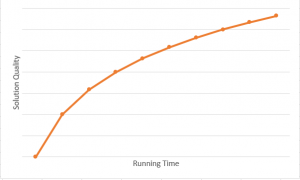Figure 1: The expected performance of anytime algorithm

## Algorithm prerequisites

Initialize: While some algorithms start with immediate guesses, anytime algorithm take more calculated approach and have a start – up period before making any guesses

Growth direction: How the quality of the program’s “output” or result, varies as run time

Growth rate: Amount of increase with each step. Does it change constantly or unpredictably

End condition: The amount of runtime needed

## Case studies

• Clustering with time series

In clustering, we must compute distance or similarity between pairs of time series and we also have a lot of measurements. As many researches proved that dynamic time warping (DTW) is more robust than others, but the complexity O(N2) of DTW make computation more trouble.

With anytime algorithm, the life is easier than ever. Below is pseudocode for anytime clustering algorithm.

Algorithm [Clusters] = AnytimeClustering(Dataset)
1.  aDTW = BuildApproDistMatrix(Dataset)
2.  Clusters = Clustering(aDTW, Dataset)
3.  Disp("Setup is done, interruption is possible")
4.  O = OrderToUpdateDTW(Dataset)
5.  For i = 1:Length(O)
6.     aDTW(O(i)) = DTW(Dataset, O(i))
7.     if UserInterruptIsTrue()
8.        Clusters = Clustering(aDTW, Dataset)
9.        if UserTerminateIsTrue()
10.          return
11.       endif
12.    endif
13. endfor
14. Clusters = Clustering(aDTW, Dataset)

Firstly, we need to approximate the distance matrix of dataset.

Secondly, we do cluster to get the very basic result.

Thirdly, we make some heuristic to find the optimal solution to update the distance matrix, after each updating, we get a better result than before.

Lastly, if the algorithm keeps running longer, it will finish the task and we can get the final optimal output.

• Learning optimal Bayesian networks

Another common example for anytime algorithm is searching optimal output in Bayesian networks. As we know that Bayesian networks is NP – hard. If we have too many variables or events, the algorithm will fail due to limited time and memory. That’s why anytime weighted A* algorithm is often applied to find an optimal result.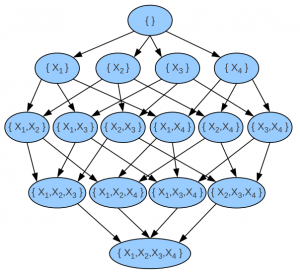Figure 2: Bayesian Networks of 4 variables

Weighted A* algorithm use this cost function to minimize

f(n) = g(n) + ε * h(n)

where n is the last node on the graph, g(n) is the cost of the path from the start node to n and h(n) is a heuristic that estimates the cost of the cheapest path from n to the last node, ε gradually lowers to 1. The anytime algorithm bases on this cost function to stop and continue at any time.

## Overfitting in Machine Learning

In any data science project, once the variables that correspond to business objectives are defined, typically the project proceeds with the following aims: (1) to understand the data quantitatively, (2) to describe the data by producing a ‘model’ that represents adequately the data at hand, and (3) to use this model to predict the outcome of those variables from ‘future’ observed data.

Anyone dealing with machine learning is bound to be familiar with the phenomenon of overfitting, one of the first things taught to students, and probably one of the problems that will continue to shadow us in any data-centric job: when the predictive model works very well in the lab, but behaves poorly when deployed in the real world.

## What is Overfitting?

Overfitting is defined as “the production of an analysis which corresponds too closely or exactly to a particular set of data, and may therefore fail to fit additional data or predict future observations reliably.”

Whether a regression, decision tree, or neural network, the different models are constructed differently: some depend on a few features (in the case of a linear model), some are too complex to visualize and understand at a glance (in the case of a deep neural network). Since there is inherently noise in data, the model fitting (‘learning’) process can either learn too little pattern (‘underfit’), or learn too much ‘false’ patterns discernible in the ‘noise’ of the seen data (‘overfit’) not meant to be present in the intended application, that end up distorting the model. Typically, this can be illustrated in the diagram below:## Detecting overfitting

In Machine Learning (ML), training and testing are often done on different parts of data available. The models — whether they be trees, neural networks, equations — that have characterized the training data very well, are validated on a ‘different’ part of data. Overfitting is detected when the model has learned too much ‘false patterns’ from the training data that do not generalize to the validation data. As such, most ML practitioners reduce the problem of overfitting to a matter of knowing when to stop training, typically by choosing an ‘early stopping’ value around the red dotted line, illustrated in the loss vs. number of iterations graph below.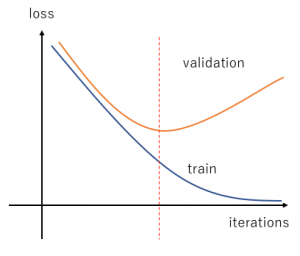If detecting overfitting is reduced to a matter of “learning enough”, inherent to the contemporary ML training process, it is assumed to be “solved” when the model works on a portion of the held-out data. In some cases, k-fold / cross-validation is performed, which basically splits the data into k ‘folds’: one held-out test set and k-1 training sets. Given that most models do not make it to the crucible of production environment, most practitioners stop at demonstrating (in a lab-controlled environment) that the model “works”; when the numbers from cross-validation statistics check out fine, showing consistent performance across all folds.

## But.. is it so simple?

In simpler or more trivial models, overfitting may not even be noticeable! However, with more rigorous testing or when the model is lucky enough to be deployed to production stage, there is usually more than one validation set (or more than one client’s data!). It is not infrequent that we see various validation/real-world data do not ‘converge’ at the same rate, making the task of choosing early stopping point more challenging. With just two different validation data sets, one can observe the following: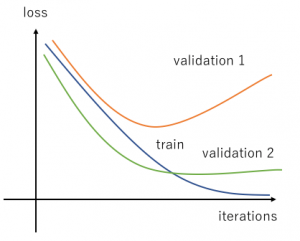What about this curve?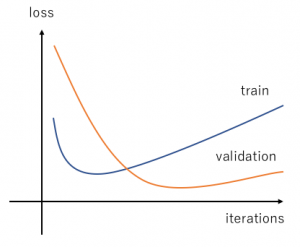When we get curves that look like the graphs above, it is harder to answer these questions: When does overfitting ‘begin’? How to deal with multiple loss functions? What is happening here??

## Digging your way out

Textbook ML prescribes many ‘solutions’ — which might just work if you are lucky — sometimes without you knowing exactly what is the problem:

• Data augmentation:
Deep down, most overfitting problems will go away if we have abundant amount of ‘good enough’ data, which for most data scientists is a utopian dream. Limited resource makes it impossible to collect the perfect data: clean, complete, unbiased, independent and cheap. For many neural network ML pipelines, data augmentation has become de rigeur, which aim to make the model not learn characteristics unique to the dataset, by multiplying the amount of training data available. Without collecting more data, data can be ‘multiplied’ by varying them through small perturbations to make them seem different to the model. Whether or not this approach is effective will depend on what is your model learning!
• Regularization:
Regularization term is routinely added in model fitting, to penalize overly complex models. In regression methods, L1 or L2 penalty terms are often added to encourage smaller coefficients and thus, ‘simpler’ models. In decision trees, methods such as decision tree pruning or limiting tree maximum depth, are typical ways to ‘keep model simple’. Combined with ensemble methods, e.g. bagging or boosting, they can be used to avoid overfitting and make use of multiple weak learners to arrive  at a robust predictor. In DL techniques, regularization is achieved by introducing dropout layer, that will randomly turn off neurons during training.

If you have gone through all those steps outlined above and still feel somehow that you just got lucky with a particular training/validation data set, you are not alone. We need to dig deeper in order to truly understand what is happening and get out of this sticky mess, or accept the risk of deploying such limited model and set up future trap for ourselves!

## Introduction

Autoencoders provide a powerful framework for learning compressed representations by encoding all of the information needed to reconstruct a data point in a latent code. In some cases, autoencoders can “interpolate”: By decoding the convex combination of the latent codes for two datapoints, the autoencoder can produce an output which semantically mixes characteristics from the datapoints. In this paper, we propose a regularization procedure which encourages interpolated outputs to appear more realistic by fooling a critic network which has been trained to recover the mixing coefficient from interpolated data. We then develop a simple benchmark task where we can quantitatively measure the extent to which various autoencoders can interpolate and show that our regularizer dramatically improves interpolation in this setting. We also demonstrate empirically that our regularizer produces latent codes which are more effective on downstream tasks, suggesting a possible link between interpolation abilities and learning useful representations. – 

The idea comes from the paper “Implementation from paper: Understanding and Improving Interpolation in Autoencoders via an Adversarial Regularizer” (https://arxiv.org/abs/1807.07543), also known as ACAI framework.

Today I will walk through the implementation of this fantastic idea. The implementation is based on tensorflow 2.0 and python 3.6. Let’s start!

## Implementation

First, we need to import some dependency packages.

import tensorflow as tf
from tensorflow import keras
from tensorflow.keras.datasets import mnist
from tensorflow.keras.layers import Input, Dense, Reshape, Flatten, Dropout, multiply, GaussianNoise
from tensorflow.keras.layers import BatchNormalization, Activation, Embedding, ZeroPadding2D
from tensorflow.keras.layers import MaxPooling2D
from tensorflow.keras.layers import LeakyReLU
from tensorflow.keras.layers import UpSampling2D, Conv2D, Reshape
from tensorflow.keras.layers import Lambda
from tensorflow.keras.models import Sequential, Model
from tensorflow.keras.optimizers import Adam
from tensorflow.keras import losses
from tensorflow.keras.utils import to_categorical
from tensorflow.keras.datasets import mnist
import keras.backend as K

import matplotlib.pyplot as plt

import numpy as np
import tqdm
import os

import io
from PIL
import Image
from sklearn.decomposition import PCA
# from sklearn.manifold import TSNE
import seaborn as sns
import pandas as pd


Next, we define the overall framework of ACAI, which composes of 3 parts: encoder, decoder and discriminator (also called as critic in the paper).

class ACAI():
def __init__(self, img_shape=(28,28), latent_dim=32, disc_reg_coef=0.2, ae_reg_coef=0.5, dropout=0.0):
self.latent_dim = latent_dim
self.ae_optim = Adam(0.0001)
self.d_optim = Adam(0.0001)
self.img_shape = img_shape
self.dropout = dropout
self.disc_reg_coef = disc_reg_coef
self.ae_reg_coef = ae_reg_coef
self.intitializer = tf.keras.initializers.VarianceScaling(
scale=0.2, mode='fan_in', distribution='truncated_normal')
self.initialize_models(self.img_shape, self.latent_dim)

def initialize_models(self, img_shape, latent_dim):
self.encoder = self.build_encoder(img_shape, latent_dim)
self.decoder = self.build_decoder(latent_dim, img_shape)
self.discriminator = self.build_discriminator(latent_dim, img_shape)

img = Input(shape=img_shape)
latent = self.encoder(img)
res_img = self.decoder(latent)

self.autoencoder = Model(img, res_img)
discri_out = self.discriminator(img)

def build_encoder(self, img_shape, latent_dim):
encoder = Sequential(name='encoder')
encoder.add(Flatten(input_shape=img_shape))
encoder.add(Dense(1000, activation=tf.nn.leaky_relu, kernel_initializer=self.intitializer))
encoder.add(Dropout(self.dropout))
encoder.add(Dense(1000, activation=tf.nn.leaky_relu, kernel_initializer=self.intitializer))
encoder.add(Dropout(self.dropout))
encoder.add(Dense(latent_dim))

encoder.summary()
return encoder

def build_decoder(self, latent_dim, img_shape):
decoder = Sequential(name='decoder')
decoder.add(Dense(1000, input_dim=latent_dim, activation=tf.nn.leaky_relu, kernel_initializer=self.intitializer))
decoder.add(Dropout(self.dropout))
decoder.add(Dense(1000, activation=tf.nn.leaky_relu, kernel_initializer=self.intitializer))
decoder.add(Dropout(self.dropout))
decoder.add(Dense(np.prod(img_shape), activation='sigmoid'))
decoder.add(Reshape(img_shape))

decoder.summary()
return decoder

def build_discriminator(self, latent_dim, img_shape):
discriminator = Sequential(name='discriminator')
discriminator.add(Flatten(input_shape=img_shape))
discriminator.add(Dense(1000, activation=tf.nn.leaky_relu, kernel_initializer=self.intitializer))
discriminator.add(Dropout(self.dropout))
discriminator.add(Dense(1000, activation=tf.nn.leaky_relu, kernel_initializer=self.intitializer))
discriminator.add(Dropout(self.dropout))
discriminator.add(Dense(latent_dim))

# discriminator.add(Reshape((-1,)))
discriminator.add(Lambda(lambda x: tf.reduce_mean(x, axis=1)))

discriminator.summary()
return discriminator

Some utility functions for monitoring the results:

def make_image_grid(imgs, shape, prefix, save_path, is_show=False):
# Find the implementation in below github repo

def flip_tensor(t):
# Find the implementation in below github repo

def plot_to_image(figure):
# Find the implementation in below github repo

def visualize_latent_space(x, labels, n_clusters, range_lim=(-80, 80), perplexity=40, is_save=False, save_path=None):
# Find the implementation in below github repo

Next, we define the training worker, which is called at each epoch:

@tf.function
def train_on_batch(x, y, model: ACAI):
# Randomzie interpolated coefficient alpha
alpha = tf.random.uniform((x.shape, 1), 0, 1)
alpha = 0.5 - tf.abs(alpha - 0.5)  # Make interval [0, 0.5]

with tf.GradientTape() as ae_tape, tf.GradientTape() as d_tape:
# Constructs non-interpolated latent space and decoded input
latent = model.encoder(x, training=True)
res_x = model.decoder(latent, training=True)

ae_loss = tf.reduce_mean(tf.losses.mean_squared_error(tf.reshape(x, (x.shape, -1)), tf.reshape(res_x, (res_x.shape, -1))))

inp_latent = alpha * latent + (1 - alpha) * latent[::-1]
res_x_hat = model.decoder(inp_latent, training=False)

pred_alpha = model.discriminator(res_x_hat, training=True)
# pred_alpha = K.mean(pred_alpha, [1,2,3])
temp = model.discriminator(res_x + model.disc_reg_coef * (x - res_x), training=True)
# temp = K.mean(temp, [1,2,3])
disc_loss_term_1 = tf.reduce_mean(tf.square(pred_alpha - alpha))
disc_loss_term_2 = tf.reduce_mean(tf.square(temp))

reg_ae_loss = model.ae_reg_coef * tf.reduce_mean(tf.square(pred_alpha))

total_ae_loss = ae_loss + reg_ae_loss
total_d_loss = disc_loss_term_1 + disc_loss_term_2

grad_ae = ae_tape.gradient(total_ae_loss, model.autoencoder.trainable_variables)
grad_d = d_tape.gradient(total_d_loss, model.discriminator.trainable_variables)

model.ae_optim.apply_gradients(zip(grad_ae, model.autoencoder.trainable_variables))
model.d_optim.apply_gradients(zip(grad_d, model.discriminator.trainable_variables))

return {
'res_ae_loss': ae_loss,
'reg_ae_loss': reg_ae_loss,
'disc_loss': disc_loss_term_1,
'reg_disc_loss': disc_loss_term_2

}

Next, we need to define a main training function:

def train(model: ACAI, x_train, y_train, x_test,
batch_size, epochs=1000, save_interval=200,
save_path='./images'):
n_epochs = tqdm.tqdm_notebook(range(epochs))
total_batches = x_train.shape // batch_size
if not os.path.exists(save_path):
os.makedirs(save_path)
for epoch in n_epochs:
offset = 0
losses = []
random_idx = np.random.randint(0, x_train.shape, x_train.shape)
for batch_iter in range(total_batches):
# Randomly choose each half batch
imgs = x_train[offset:offset + batch_size,::] if (batch_iter < (total_batches - 1)) else x_train[offset:,::]
offset += batch_size

loss = train_on_batch(imgs, None, model)
losses.append(loss)

avg_loss = avg_losses(losses)
# wandb.log({'losses': avg_loss})

if epoch % save_interval == 0 or (epoch == epochs - 1):
sampled_imgs = model.autoencoder(x_test[:100])
res_img = make_image_grid(sampled_imgs.numpy(), (28,28), str(epoch), save_path)

latent = model.encoder(x_train, training=False).numpy()
latent_space_img = visualize_latent_space(latent, y_train, 10, is_save=True, save_path=f'./latent_space/{epoch}.png')
# wandb.log({'res_test_img': [wandb.Image(res_img, caption="Reconstructed images")],
#            'latent_space': [wandb.Image(latent_space_img, caption="Latent space")]})
(x_train, y_train), (x_test, y_test) = mnist.load_data()
x_train = x_train.astype(np.float32) / 255.
x_test = x_test.astype(np.float32) / 255.
ann = ACAI(dropout=0.5)
train(model=ann,
x_train=x_train,
y_train=y_train,
x_test=x_test,
batch_size=x_train.shape//4,
epochs=2000,
save_interval=50,
save_path='./images')

## Results

Some of the result from ACAI after finishing:

First is the visualization of MNIST dataset after encoded by the encoder, we can see that the cluster is well separated and applying downstream tasks on latent space will lead to significant improvement in comparison to raw data (such as clustering, try KMeans and check it out yourself :D).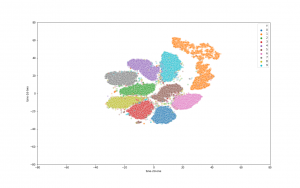Second is the visualization of interpolation power on latent space:

• Interpolation with alpha values in range [0,1.0] with step 0.1.
• 1st row and final row are source and destination image, respectively.
• Formula:
mix_latent = alpha * src_latent + (1 - alpha) * dst_latent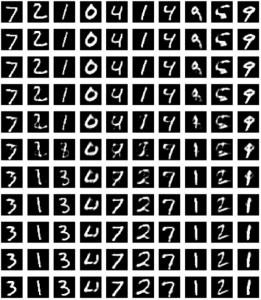We can see that there is a very smooth morphing from the digits at the top row to the digits at the bottom row.

The whole running code is available at github (acai_notebook). It’s your time to play with the paper :D.

## Reference

 David Berthelot, Colin Raffel, Aurko Roy, and Ian Goodfellow. Understanding and improving interpolation in autoencoders via an adversarial regularizer, 2018.

## Hiring Data Scientist / Engineer

We are looking for Data Scientist and Engineer.
Please check our Career Page.

## Data Science Project

Please check about experiences for Data Science Project

## Vietnam AI / Data Science LabPlease also visit Vietnam AI Lab

## Pytorch part 1: Introducing Pytorch

Pytorch is a deep learning framework and a scientific computing package
The scientific computing aspect of PyTorch is primarily a result PyTorch’s tensor library and associated tensor operations. That means you can take advantage of Pytorch for many computing tasks, thanks to its supporting tensor operation, without touching deep learning modules.

Important to note that PyTorch tensors and their associated operations are very similar to numpy n-dimensional arrays. A tensor is actually an n-dimensional array.

Pytorch build its library around Object Oriented Programming(OOP) concept. With object oriented programming, we orient our program design and structure around objects. The tensor in Pytorch is presented by the object torch.Tensor which is created from numpy ndarray objects. Two objects share memory. This makes the transition between PyTorch and NumPy very cheap from a performance perspective.

With PyTorch tensors, GPU support is built-in. It’s very easy with PyTorch to move tensors to and from a GPU if we have one installed on our system. Tensors are super important for deep learning and neural networks because they are the data structure that we ultimately use for building and training our neural networks.
Talking a bit about history.

The initial release of PyTorch was in October of 2016, and before PyTorch was created, there was and still is, another framework called Torch which is also a machine learning framework but is based on the Lua programming language. The connection between PyTorch and this Lua version, called Torch, exists because many of the developers who maintain the Lua version are the individuals who created PyTorch. And they have been working for Facebook since then till now.

Below are the primary PyTorch modules we’ll be learning about and using as we build neural networks along the way.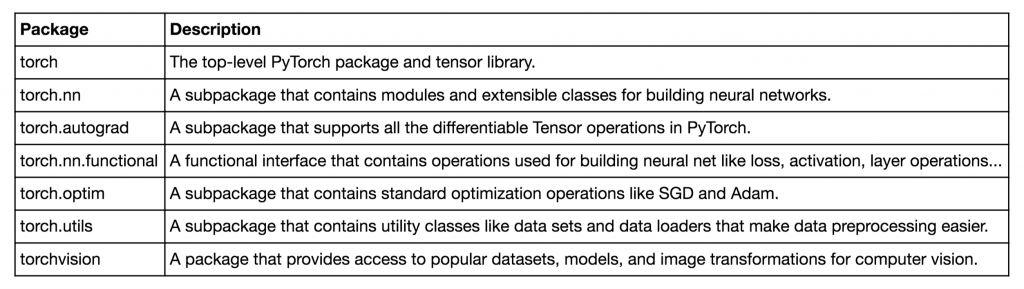Image 1. Pytorch package description

## Why use Pytorch for deep learning?

• PyTorch’s design is modern, Pythonic. When we build neural networks with PyTorch, we are super close to programming neural networks from scratch. When we write PyTorch code, we are just writing and extending standard Python classes, and when we debug PyTorch code, we are using the standard Python debugger. It’s written mostly in Python, and only drops into C++ and CUDA code for operations that are performance bottlenecks.
• It is a thin framework, which makes it more likely that PyTorch will be capable of adapting to the rapidly evolving deep learning environment as things change quickly over time.
• Stays out of the way and this makes it so that we can focus on neural networks and less on the actual framework.

## Why PyTorch is great for deep learning research

The reason for this research suitability is that Pytorch use dynamic computational graph, in contrast with tensorfow which uses static computational graph, in order to calculate derivatives.

Computational graphs are used to graph the function operations that occur on tensors inside neural networks. These graphs are then used to compute the derivatives needed to optimize the neural network. Dynamic computational graph means that the graph is generated on the fly as the operations are created. Static graphs that are fully determined before the actual operations occur.

## k-Nearest Neighbors algorithms

In this blog post, I am going to introduce one of the most intuitive algorithms in the field of Supervised Learning, the k-Nearest Neighbors algorithm (kNN).

## The original k-Nearest Neighbors algorithm

The kNN algorithm is very intuitive. Indeed, with the assumption that items close together in the dataset are typically similar, kNN infers the output of new sample by first constructing the distance score with every sample in the training dataset. From there, it creates a ‘neighbor zone’ by selecting samples that are ‘near’ the candidate one, and does the supervised tasks based on samples lie inside that zone. The task could be either classification or regression.

Let’s start with the basic kNN algorithm. Letbe our training dataset withsamples belong toclasses, whereis the class of one sample, anddenotes the corresponding feature vector that describes the characteristics of that sample. Furthermore, it is necessary to define the suitable distance metric, since it drives the algorithm to select neighbors and make prediction later on. The distance metric, is a mappingover a vector space, where the following conditions are satisfied:

••••In the following steps to describe the k-Nearest Neighbors algorithm, the Euclidean distance will be used as the distance metric.

For any new instance:

• Findwhereis the set ofsamples that are closest to• The way to define the nearest neighbors is based on distance metric(Note that we are using Euclidean distance).• The classifieris defined as:whereis the unit function. Note that for the regression problem, the functionwill just an average of all response valuesfrom neighbor samples.

## Weighted k-Nearest Neighbors

In the kNN algorithm, we weight all neighbors equally. It may affect the inference steps, especially when the neighbor zone becomes bigger and bigger. To strengthen the effect of ‘close’ neighbors than others, the weighted scheme of k-Nearest Neighbors is applied.

Weighted k-Nearest Neighbors is based on the idea that, within, such observations that are closer to, should get a higher weight than the further neighbors. Now it is necessary to note some properties of any weighting schemeson any distance metric:

•••decreases monotonously forFor any new instance:

• We findwhereis the set ofsamples that are closest to• Theth neighbor is used for standardization of thesmallest distance:• We transform the standardized distancewith any kernel functioninto weights.
• The classifieris defined as:## The pros and cons of kNN, and further topics

The kNN and weighted kNN do not rely on any specific assumption on the distribution of the data, so it is quite easy to apply it on many problems as the baseline model. Furthermore, kNN (and its family) is very intuitive for understanding and implementing, which again make it a worthy try-it-first approach for many supervised problems.

Despite those facts, kNN still has challenges in some aspects: It is computationally expensive – especially when the dataset size becomes huge. Another challenge is choosing the ‘correct’ distance metric that best follows the assumption for using this algorithm: items close together in the data set should be typically similar. Lastly, the curse of dimensionality heavily affects the distance metric. Beyer et al. proves that, under some preconditions, in high dimension space, all points converge to the same distance from the query point. In this case, the concept of ‘nearest neighbors’ is no longer meaningful.

## Machine Learning development with AWS Sage Maker

Make your Machine Learning team working easier, focus more on business and quick deployment with AWS managed service SageMaker.

Today, Machine Learning(ML) is resolving complex problems which make more business values for customer and many companies also apply ML to resolve robust business problems. ML have more benefit, but also more challenges to building the ML model with high accuracy. I currently working on the AI team to help the company deliver AI/ML project quickly and help Data Scientist(DS) team developing Data Pipeline, Machine Learning pipeline which helps project grow and quick delivery with high quality.

## Overview of Machine Learning development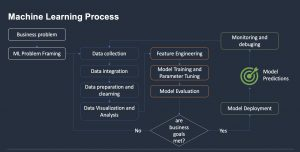Figure1. Machine learning process

Here is the basic machine learning process which is the practice model of big companies. We are including multiple phases (business analyst, data processing, model training and deployment), multiple steps each phase and a fleet of tools that we use to result in dedicated steps.

Business problems: is including problems that challenge the business and we can use ML learning as a better solution to resolve it.
ML Problem Framing: is phase help DS and Engineering definition for ML problems, propose ML solutions, data pipeline and planing.
Data processing(Collection, integration, preparation and cleaning, visualization and analysis): This phase including multiple steps that help to prepare data for visualization and ML training.
Model Training(Feature engineering, Model training and parameter tuning, model evaluation): DS and developer will working on this phase to develop engineering features and prepare data for specific model and training model using framework such as Tensorflow, Pytorch.

When we don’t use any platform such as AWS SageMaker or Azure ML Studio then we take more time to develop with complex stack skills. We need to have many skills in compute, network, storage, ML frameworks, programming language, engineering features …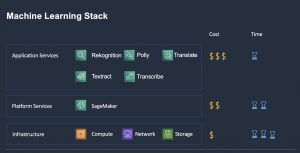Actually, when we develop an ML model with a lack of skills and complex components that take more time to handle tasks about programming, compute and challenges for the engineering team which using the model and deploy it. In Figure 2, we have multiple instants of cloud computing (Infrastructure as a ServicePlatform as a ServiceApplication as a Service) that provide resource type according to business necessary at the level of control. We can choose a specific instant layer for business necessary or compact of layers to meet the business objective. So, which Machine Learning projects and research environment, I would like to highly recommend for any DS and Develop should use PaaS and AaaS first to meet your business requirement first to quick delivery, reduce cost and effort. That is the main reason I want to describe AWS SageMaker service which can use as a standard platform for ML team to quickly develop/deploy ML model, focus on resolve ML business problems and improve the quality of the model.

## AWS SageMaker offers the base of end to end machine learning development environment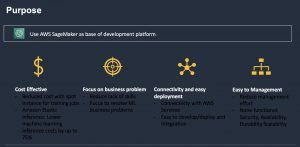Figure 3. AWS SageMaker benefits

When we developing an ML model, we need to take care of multiple partitions and sometimes we want to try with a new model and responsibility accuracy response as quickly as possible. So, that also depends on “Is there enough data for processing and training?” and “How many time will take for training a new model?”. AWS SageMaker is using in thousands of AI companies and developed by experts and best practices of ML which help to improve the ML process, working environment. In my opinion, when I want to focus on building a model and resolve the challenge problem then I want to make another easy at the basic level and spend more time to resolve the main problems at first. SageMaker provides me a notebook solution and a notebook is a great space that I coding and analyzing data for training. Working together with SageMaker SDK, I can easy to connect and use other resources inside of AWS such as S3 bucket and training jobs. Everything helps me quickly develop and deliver a new model. So, I want to highlight the main benefit which we got from this service and also have disadvantages.

Advantages
*💰 Cost-effective:

– SageMaker provide solution for training ML model with training jobs that are distributed, elastic, high-performance computing using spot instance to save cost up to 90%, pay only for training time in seconds. (document)
– Elastic Inference: This feature help to save cost for computes need GPU to processing deep learning model such as prediction time. (document)
* 🎯 Reduce lack of skills and focus to resolve business problems. We can easy to setup training environment from notebook with “click” for elastic of CPUs/GPUs
* 🌐 Connectivity and easy to deploy
– AWS SageMaker is AWS manged service and it easy to integrate with other AWS services inside of private network. Which also impact to big data solution, ETL processing with data can be processing inside of private network and reduce cost for transfer.
– EndPoint function help DS/Develop easy to deploy trained model with “clicks” or from SDK. (document)
* 🏢 Easy to management: When working with multiple teams on AWS, more resource will come everyday and challenges with IT team to manage resources, roles which will impact to cost and security. AWS manged service will help to reduce resource we need to create.

Disadvantages
* AWS SageMaker is a managed service, it implements with best practices and focuses on popular frameworks, sometimes it does not match your requirement and should be considered before choosing it.
* 🎿 Learning new skills and basic knowledge of AWS Cloud: When we working on AWS cloud, basic knowledge about cloud infrastructure is necessary and new knowledge with AWS managed service which you want to use.
* 👮 It also expensive than normal EC2 instance because it supports dedicated for ML, we need to choose the right resource for the development to save cost.

AWS SageMaker is the best suite service for the production environment. It helps to build a quality model and standard environment. Which reduces the risk in product development. We trade-off to get most of the benefit and quickly to achieve the goal of the team. Thank you so much for reading, please let me know if you have any concerns.

## I. A Brief Overview

Consider an example of a courtroom trial:

A car company C is accused of not manufacturing environment-friendly vehicles. The average CO2 emission per car from different manufacturers based on a survey from the previous year is 120.4 grams per kilometer. But for a random batch of 100 cars produced at C’s factory, the average CO2 emission is 121.2 grams per kilometer with a standard deviation 1.8.

At the trial, Company C is not considered to be guilty as long as their wrongdoing is not proven. A public prosecutor tries to prove that C is guilty and can only succeed when enough evidence is presented.

The example above illustrates the concepts of hypothesis testing; specifically, there are two conflicting hypotheses:

i) C is not guilty; or

ii) C is guilty

The first is called the null hypothesis (denoted by H0), and the second the alternative hypothesis (denoted by HA). At the start of the trial the null hypothesis is temporarily accepted, until proven otherwise. The goal of hypothesis testing is to perform some sort of transformed comparison between the two numbers 121.2 and 120.4 to either reject H0 and accept HA or vice versa. This is a one-sample mean testing because we are comparing the average value obtained from one sample (121.2) with the average value assumed to represent for the whole population (120.4)

## II. Required Steps for Hypothesis Testing

The six steps below must be followed to conduct a hypothesis test. The details will be elaborated with our example afterwards.

1) Set up null and alternative hypotheses and check conditions.

2) Determine the significance level, alpha.

3) Calculate the test statistic.

4) Calculate the probability value (a.k.a the p-value), or find the rejection region. For the following example, we will use the p-value.

5) Make a decision about the null hypothesis.

6) State the overall conclusion.

## III. A step-by-step example

### 1) Set up hypotheses:

We already mentioned in the beginning the two hypotheses. But now we will formalize them:

Null hypothesis:

Company C’s CO2 mean (denoted by μ ) is equal to the population mean (denoted by μ0):           μ = μ0

Alternative hypothesis:

Company C’s CO2 mean is greater than the population mean: μ > μ0

The hypothesis testing for one-sample mean we are conducting requires the data to come from an approximately normal distribution or a large enough sample size, which can be quite subjective. To keep things simple, we decide that the data gathered from company C is big enough with the sample size being 100 cars.

### 2) Determine the significance level, alpha, or confidence level

The significance level and its complementary, the confidence level, provide a level of probability cutoff for our test to make decisions about the hypotheses. A common value for alpha is 5%, which is the same as a confidence level of 95%.

### 3) Calculate the test statistic

For one-sample mean test, we calculate the t* test statistic using the formula: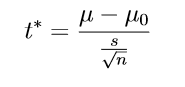where s is the standard deviation from the sample we are testing, 1.8, and n is the size of the sample, 100.

## Bias in Data Science – the Good, the Bad and the Avoidable !?

In recent years, there have been a few prominent examples of accidental bias in machine-learning applications, such as smart phones’ beauty filters (that essentially ended up whitening skin)  or Microsoft’s from-innocent-teen-to-racist-in-24-hours chatbot [2,3]. Examples such as these obviously fell victim to inherently biased data being fed into algorithms too complex to allow for much transparency. Hidden bias continues to be an issue on ubiquitous social media platforms, such as Instagram, whose curators appear to profess themselves both regretful AND baffled . Unfortunately, any model will somewhat regurgitate what it has been fed and interventions at this level of model complexity may prove tricky.

Interestingly, bias itself does not need to be harmful and is often built into a model’s design on purpose, either to address only a subset of the overall population or to model a real-world state, for instance when predicting house prices from its size and number of bedrooms, the model’s bias parameter often represents the average house price in the data set. Thus, we need to distinguish between conscious and unconscious bias in data analysis. Additionally, there is the factor of intent, i.e. is the person conducting the analysis well-intentioned to follow good scientific method or trying to manipulate it to achieve a particular outcome.

In the following, I will only discuss aspects of unintentional and unconscious bias, meaning bias hidden from the researcher or data scientist introducing it. This is by no means an exhaustive discussion, but merely a highlight of some pervasive aspects:

A. Data availability bias

B. Coherent theory bias

C. Method availability/popularity bias

## A. Data availability bias

A. The problem of scientists’ selecting their data out of convenience rather than suitability or representativeness for the current task has been around for a while , e.g. the ideal data set may not be available in machine-readable format or would require higher costs and more time for processing, in short, several obstacles to doing an analysis quickly. For instance, in the area of Natural Language Processing, the major European languages, like English, French and German etc. tend to receive more attention because both data and tools to analyze them are widely available. Similarly, psychology research has mostly focused on so-called WEIRD societies (White, Educated, Industrialized, Rich, Democratic)  and out of convenience often targets the even smaller population of “North American college students” that unsurprisingly have been found to not represent human populations at large.

## B. Coherent theory bias

B. Various studies suggest that we as people strongly favour truths that fit into our pre-existent world view, and why would scientists be exempt from this? Thus, it appears when people analyze data they are often biased by their underlying beliefs about the outcome and are then less likely to yield to unexpected non-significant results . This does not include scientists disregarding new evidence because of conflicting interests . This phenomenon is commonly referred to as confirmation bias or, more fittingly, “myside” bias.

## C. Method availability/popularity bias

C. There is a tendency of hailing new trendy algorithms as one-fits-all solutions for whatever task or application. The solution is presented before examining the problem and its actual requirements. While more complex models are often more powerful, this comes at the cost of interpretability, which in some cases is not advisable. Additionally, some methods, both simple and complex ones, enjoy popularity primarily because they come ready-to-use in one’s favourite programming language.

Going forward…

We as data scientists should:

a. Select our data carefully with our objective in mind. Get to know our data and its limitations.

b. Be honest with ourselves about possible emotional investment in our analyses’ outcomes and resulting conflicts.

c. Examine the problem and its (theoretical) solutions BEFORE making any model design choices.

## References:

 https://www.bbc.com/news/technology-35902104(last accessed 21.10.2020)

 Joseph Rudman (2003) Cherry Picking in Nontraditional Authorship Attribution Studies, CHANCE, 16:2,26-32, DOI: 10.1080/09332480.2003.10554845

 Henrich, Joseph; Heine, Steven J., and Norenzayan, Ara. The Weirdest People in the World? Behavioral and Brain Sciences, 33(2-3):61–83, 2010. doi: 10.1017/S0140525X0999152X.

 Hewitt CE, Mitchell N, Torgerson DJ. Heed the data when results are not significant. BMJ. 2008;336(7634):23-25. doi:10.1136/bmj.39379.359560.AD

 Ioannidis JP. Why most published research findings are false. PLoS Med. 2005;2(8):e124. doi:10.1371/journal.pmed.0020124

## Hiring Data Scientist / Engineer

We are looking for Data Scientist and Engineer.
Please check our Career Page.

## Data Science Project

Please check about experiences for Data Science Project

## Vietnam AI / Data Science LabPlease also visit Vietnam AI Lab

## Motivation

What makes computers useful for us is primarily the ability to solve problems. The procedure in which computers solve a problem is an algorithm.  In the recent context of increasing number of algorithms available for solving data-related problems, there is increasing demand for higher level of understanding of algorithm’s performance in order for data scientists to choose the right algorithms for their problems.

Having a general perception for efficiency of an algorithm would help shaping the thought process for creating or choosing better algorithms. With this intention in mind, I would like to create a series of posts to discuss about what makes a good algorithm in practice, or in short, efficient algorithm. And this article is the first step of the journey.

## Define Efficiency

An algorithm is considered efficient if its resource consumption, also known as computational cost, is at or below some acceptable level. Roughly speaking, ‘acceptable’ means: it will run in a reasonable amount of time or space on an available computer, typically as a function of the size of the input.

There are many ways in which the resources used by an algorithm can be measured: the two most common measures are speed and memory usage. In the next 2 sections, we will be looking at the two different perspectives for measuring efficiency of an algorithm from theoretician and practitioners.

## Theoreticians perspective

Theoreticians are interested in measuring efficiency of algorithm without actually have to run it in several machines and input size. The key idea is that they are not going to actually consider the runtimes of the algorithm on any particular input. Rather, they look at what are known as asymptotic runtimes. Or in other words, they look at how the runtime scale with input size (n) as n gets larger. Does the output scale proportional to n, or proportional to n squared, or maybe exponential in n? In fact, these rate of growth are so different that as long as n is sufficiently large, constant multiples that come from other measures like temporary disk usage, long-term disk usage would be relatively small and neglected.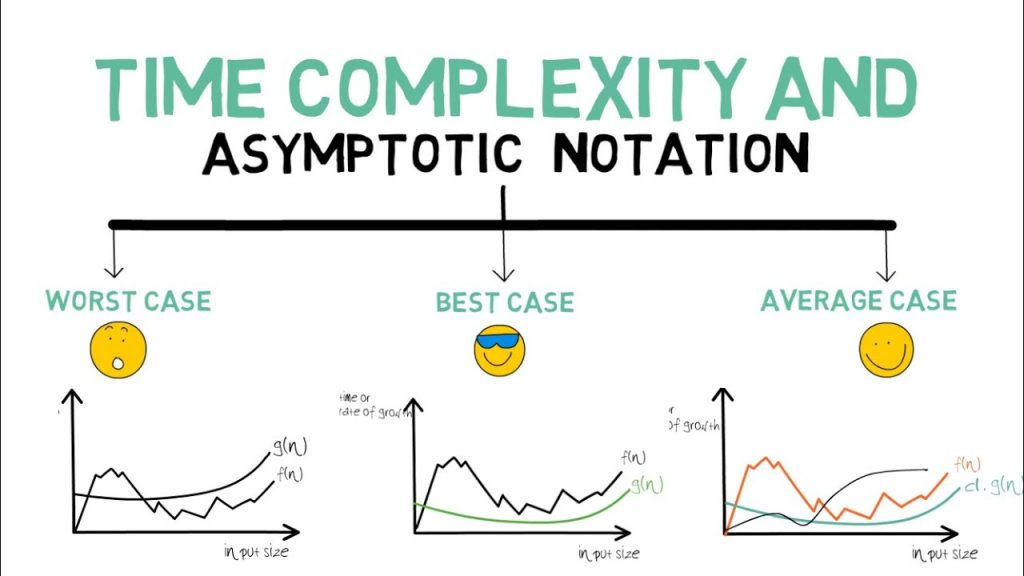Fig1:An illustration for time complexity using asymptotic notation for different functions

## Practitioner perspective

While certainly useful, the asymptotic runtime of an algorithm doesn’t tell the whole story. There are algorithms that have good asymptotic runtime, but constants that are so huge that they effectively can’t be used. Ever. Lipton calls them Galactic Algorithms. A galactic algorithm is an algorithm that is wonderful in its asymptotic behavior, but is never used to actual compute anything.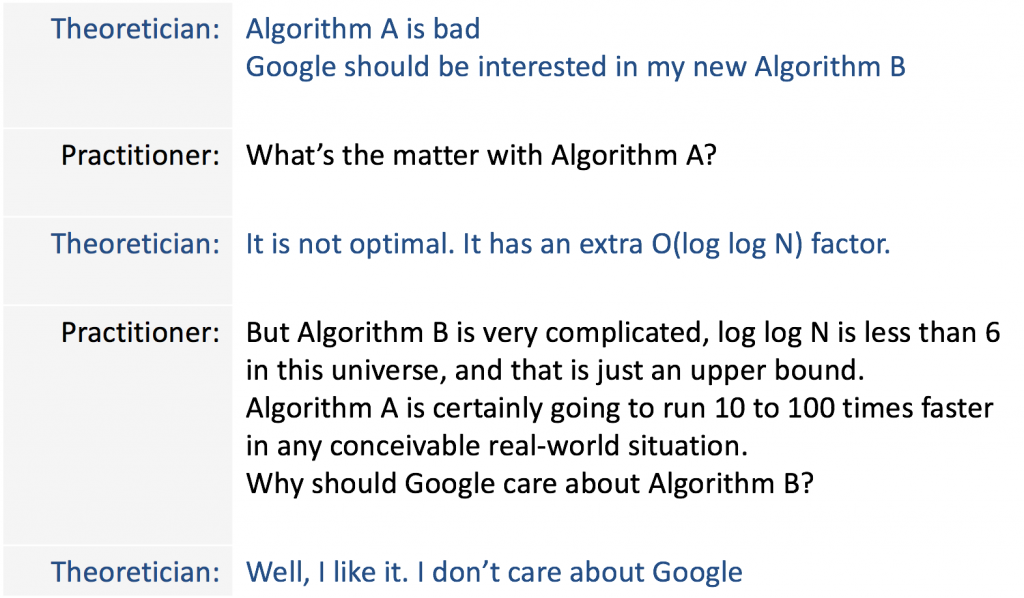Fig2: A fun exchange between a theoretician and practitioner

In practice, there are other factors which can affect the efficiency of an algorithm, such as requirements for accuracy and/or reliability. As detailed below, the way in which an algorithm is implemented can also have a significant effect on actual efficiency, though many aspects of this relate to optimization issues.

Implementation issues can also have an effect on efficiency, such as the choice of programming language, or the way in which the algorithm is actually coded, or the choice of a compiler for a particular language, or the compilation options used, or even the operating system being used. In many cases a language implemented by an interpreter may be much slower than a language implemented by a compiler.